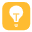240

10

188

156074A+

# 解决MyBatis的mapper中SQL小于号或大于号报错-tag name expected

解决方法很简单，我们只需要将个别特殊符号使用转义字符就好了：

 < <= > >= & ' " < <= > >= & ' "

```<if test="userId >0 && userId <= 10 ">
t.userId = #{userId}
</if>```

```<selece id="xxx" resultmap="xxx" parameterType="xxx">
SELECT
*
FROM
( SELECT ROWNUM AS rowno, t.ID FROM t_user t WHERE ROWNUM <= 2000) t_user
WHERE
t_user.rowno >= 1;(错误写法)
</select>```
```<selece id="xxx" resultmap="xxx" parameterType="xxx">
SELECT
*
FROM
( SELECT ROWNUM AS rowno, t.ID FROM t_user t WHERE ROWNUM <= 2000) t_user
WHERE
t_user.rowno &gt;=1;(正确写法)
</select>```

••友情提示 请保留版权标识

java
30%
javascript
55%
c
10%